Learning Library

# Writing Subtraction Equations

How do your kid's subtraction skills stack up? His academic confidence will soar to new heights after practicing some two-digit subtraction!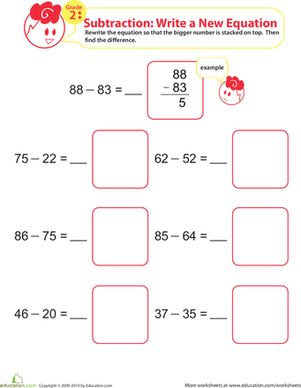### Subtraction Without Regrouping

This printable math worksheet asks your second grader to rewrite each subtraction equation, stacking the larger number on top, and write down each answer.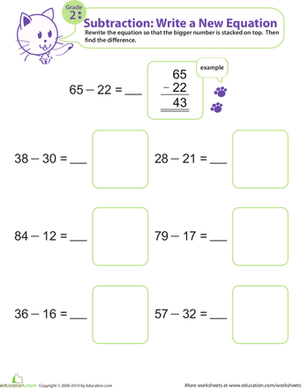### Writing Subtraction Equations 2

Provide your second grader with a healthy subtraction boost with this kitten-adorned worksheet. She will be practicing subtraction in no time!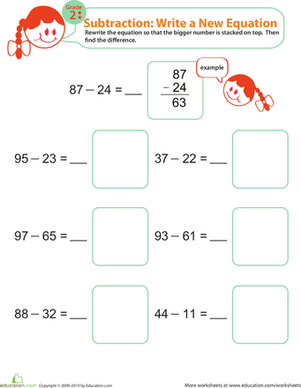### Writing Subtraction Equations 3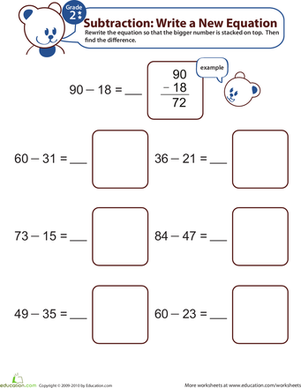### Writing Subtraction Equations 4

This printable page of subtraction problems will give your second grader invaluable two-digit subtraction practice and a familiarity with writing equations.### Writing Subtraction Equations 5

Your child will rewrite each equation according to the directions, then find the answers. Your child will practice solving problems and writing equations too!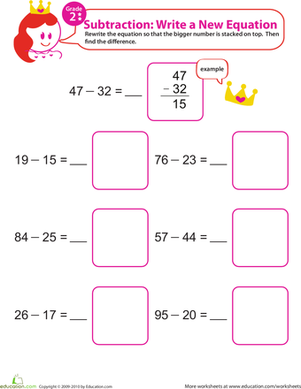### Writing Subtraction Equations 6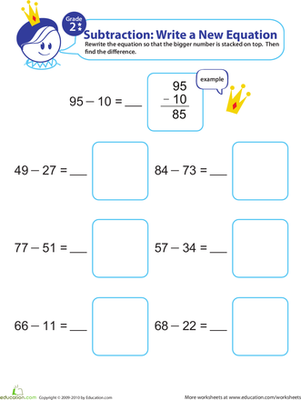### Writing Subtraction Equations 7

Help your kid perfect his subtraction skills with this math worksheet. Your kid will get subtraction practice and learn to express equations in different ways.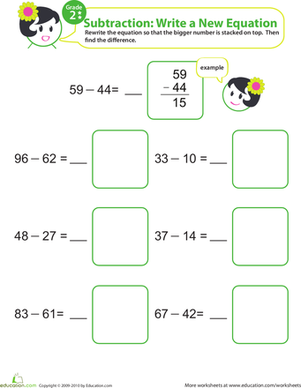### Writing Subtraction Equations 8

Two-digit subtraction can be the bane of a second grader's existence. Encourage your child to do some extra practice at home with this adorable printable.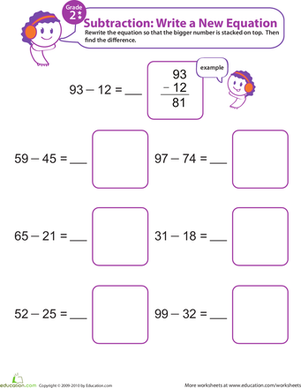### Writing Subtraction Equations 9

Give your kid a boost in math with this fun subtraction worksheet. For each problem, your kid will rewrite the equation a different way and find the difference.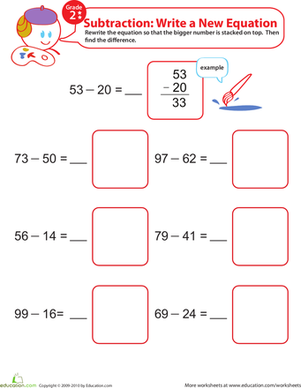### Writing Subtraction Equations 10

Mr. Artist needs to solve a set of subtraction problems, but he has trouble understanding these equations as they are written. Will your child give him a hand?

Create new collection

0

### New Collection>

0 items

What could we do to improve Education.com?